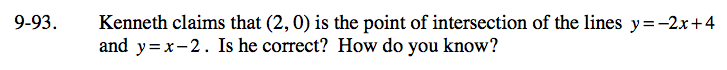Home > ACC7 > Chapter cc39 > Lesson cc39.2.3 > Problem9-93

9-93.

Kenneth claims that (2, 0) is the point of intersection of the lines y = –2x + 4 and y = x − 2. Is he correct? How do you know? Homework Help ✎A point of intersection means that a certain point makes both equations true.

Substitute Kenneth's point into both equations. Does it make them both true?